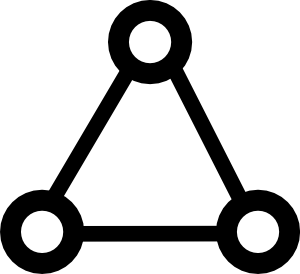# Math Insight

### Small world networks

Two properties of many real world networks are that the distance between any pairs of nodes is relatively small while at the same time the level of transitivity, or clustering is relatively high.

Erdös-Rényi random networks (ER random networks) do have a low average path length, meaning that there tends to be a path between a pair of nodes that involves only a few edges. This property is shared by many real world networks, and is often called the small world property. This notion has been popularized by terms like the “six degrees of separation“ between any two people, meaning they are typically connected by a chain of six or fewer edges in a social network graph where people are viewed as nodes and acquaintances are linked by an edge in the graph.

However, one property of real world graphs, such as social networks, that is absent from ER random graphs is presence of a large degree of transitivity. Since your friends are likely to be friends, the social network describing these friendship is likely to have many more triangles than predicted by the ER random network model.One way to increase transitivity is to arrange all the nodes in a lattice. The simplest example is to arrange the nodes in a long row, even connecting the ends to form a circle so that one doesn't have to worry about boundaries. Then, if one connects each node just to the nodes that are nearby, transitivity in the resulting network naturally arises. Since a pair of nodes that are connected to a third node will tend to be close to each in the lattice, it is likely they are also connected. The number of triangles in the graph will be relatively high, resulting in high transitivity or clustering.

However, such a lattice model will not have the small world property. Since all connections are two nearby nodes, it will take a long chain of connections to reach a node that is large distance away on the lattice. If the nodes are arranged on a circle, then the shortest path between nodes at opposites of the circle will be quite large.

Watt and Strogatz developed a model that combines the transitivity of the lattice model with the low path length of the random network model, creating a model known as the small world network.1 They start with the lattice model that has high clustering and high mean path length. Then, the add to the model a probability $p$ that an edge is rewired, meaning that the edge is disconnected from one of its nodes and then randomly connected to another node anywhere in the network. Each edge is chosen to be rewired independent with probability $p$.

When the probability $p$ is low, then most connections are still the original local connections that they connect nodes that are nearby in the lattice. For this reason, the transitivity is still high. However, some of the edges that have been rewired might turn into long distance connections that connect nodes that are far away from each other in the lattice. These long distance connections, or shortcuts, immediately create a short distance between the nodes around either end of the shortcut. Implicit in this conclusion is the fact that path length counts each edge as length one, so the shortcut connections add only one to the path length even though we frequently draw them as long connections.

The below applet illustrates the properties of the small world network. As you change the rewiring probability $p$, a sample network is shown as well as the mean path length $\ell$ and the clustering coefficient $C$.

Small world model network. A network of $N=200$ nodes spread around a ring. Originally, each node was symmetrically connected to its 8 nearest neighbors along the ring. But then, each edge was rewired with probability $p$. If an edge was selected for rewiring, one end of the edge was disconnected from a node and reconnected with a randomly chosen node. The rewiring creates shortcuts across the network and rapidly decreases the mean path length $l$ across the network. As you increase $p$ (by moving the point on the slider with your mouse), the mean path length decreases rapidly, as shown at the plot on the right. The rewiring also decrease the clustering coefficient $C$, but there is a large range of $p$ where the clustering coefficient is still large and the path length is small, which is the small world network regime (shaded). The path length and clustering coefficient calculated are averages over many realizations of the network for a given $p$ (and $C$ is an approximation for large network size $N$), so they don't exactly correspond to the actual network shown at the left. In the original network with $p=0$, both measures achieve their maximums of $C=0.64$ and $\ell=12.5$.

When the rewiring probability $p$ becomes large, the network becomes similar to the ER random network model and the transitivity becomes low. But, there is a large range of $p$ where the clustering coefficient $C$ is large and the average path length $\ell$ is small. For this range of the parameter $p$, the network is considered a small world network.